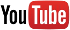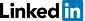Terms of serviceBrokking .net Let's keep it simpleHome
TL-blog
 DIY multicopter / RPAS flight controller          YMFC-32 - The STM32 Arduino quadcopter          YMFC-32 - The STM32 Arduino autonomous quadcopter          YMFC-AL - The self-leveling Arduino quadcopter          YABR - The Arduino Balancing Robot          Building an electric skateboard          Automated reflow hot plate          Building the Stepcraft 420
ProjectsArticlesDonationBrokking.net - Project YMFC-32 - The STM32 quadcopter - Q & A.

# Project YMFC-32 - The STM32 quadcopter - Q & A.

Click on a question to expand / collapse the answer.

This is normal. After starting the quadcopter the PID controllers and the IMU directly start to work. This means that the quadcopter tries to level itself directly after the start.

When the quadcopter is started at a (slight) tilted surface the rpm of the lower motors will increase and the rpm of the higher motors will decrease.

Always take off directly after you start the quadcopter.

If your motors run different direct on startup the ESC's are not calibrated correctly and you need to recalibrate all the ESC's with the ESC calibration sketch.

After calibration use the ESC calibration program and send a 5 as described in the setup video to check if the motors run at the same speed when the throttle is increased.

You can use the subtrims on your transmitter to level the YMFC-AL quadcopter.

Please understand that an auto-level feature is not the same as position hold. The quadcopter will always drift due to wind, turbulence, etc. No matter how level the quadcopter is.

In the original code the maximum angle is set to approximately 33 degrees. The angle is calculated as follows:

500 - 8 / 15 = 32.8 degrees

Where:
500 = standardized stick travel value
15 = original angle setting

To change the maximum angle you need to change the value 15 in the following lines:

13 = 37 degrees
14 = 35 degrees
15 = 33 degrees
16 = 31 degrees
17 = 29 degrees
18 = 27 degrees

Adjust the maximum angle with small steps to see if the quadcopter keeps performing normal.